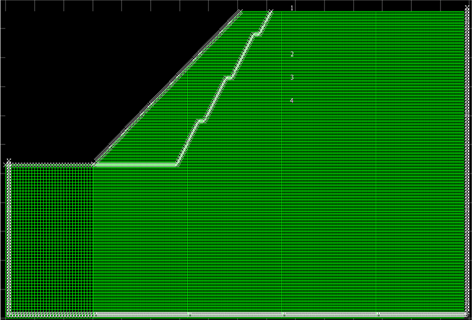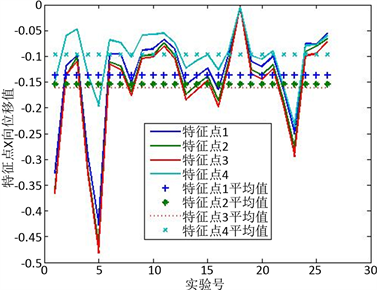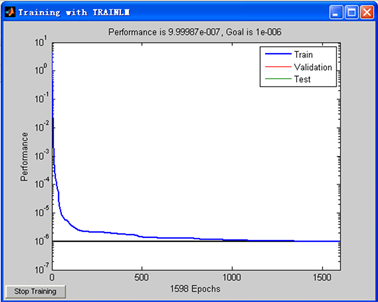﻿ 基于人工神经网络的边坡岩体力学参数反演

# 基于人工神经网络的边坡岩体力学参数反演Mechanical Parameter Inversion of Slope Rock Mass Based on Artificial Neural Network

Abstract: Based on FLAC numerical simulation experiment and in-situ deformation monitoring, simulation experiment scheme was designed according to uniform test. The artificial neural network algorithm was used for rock slope displacement back analysis, and four important mechanical parameters of the rock slope were obtained. Further, by analyzing the mechanic parameters inversion, the slope rock mass stability could be better predicted. The obtained mechanical parameters by back analysis were more accurate and consistent with the calculated results of FLAC because the effects of time and various excavations were taken into account. The mechanical parameters inversion of slope rock mass by artificial neural network has important reference value for slope support and parameter selection, and also has an important effect on engineering economy.

1. 引言

2. 神经网络模型

BP算法不仅有输入层节点、输出层节点，还可有1个或多个隐含层节点。对于输入信号，要先向前传播到隐含层节点，经作用函数后，再把隐节点的输出信号传播到输出节点，最后给出输出结果。节点的作用的激励函数通常选取S型函数，如

$f\left(x\right)=\frac{1}{1+{\text{e}}^{-x/Q}}$

${\text{net}}_{jk}=\underset{i}{\sum }{W}_{ij}{O}_{ik}$

$\frac{\partial {E}_{k}}{\partial {W}_{ij}}=\frac{\partial {E}_{k}}{\partial {\text{net}}_{jk}}\frac{\partial {\text{net}}_{jk}}{\partial {W}_{ij}}=\frac{\partial {E}_{k}}{\partial {\text{net}}_{jk}}{O}_{ik}={\delta }_{jk}{O}_{ik}$

${\delta }_{jk}=\frac{\partial {E}_{k}}{\partial {\stackrel{⌢}{y}}_{k}}\frac{\partial {\stackrel{⌢}{y}}_{k}}{\partial {\text{net}}_{jk}}=-\left({y}_{k}-{\stackrel{⌢}{y}}_{k}\right){f}^{\prime }\left({\text{net}}_{jk}\right)$ (式1)

$\begin{array}{l}{\delta }_{jk}=\frac{\partial {E}_{k}}{\partial {\text{net}}_{jk}}=\frac{\partial {E}_{k}}{\partial {O}_{jk}}\frac{\partial {O}_{jk}}{\partial {\text{net}}_{jk}}=\frac{\partial {E}_{k}}{\partial {O}_{jk}}{f}^{\prime }\left({\text{net}}_{jk}\right)\\ \frac{\partial {E}_{k}}{\partial {O}_{jk}}=\underset{m}{\sum }\frac{\partial {E}_{k}}{\partial {\text{net}}_{mk}}\frac{\partial {\text{net}}_{mk}}{\partial {O}_{jk}}\\ \text{\hspace{0.17em}}\text{\hspace{0.17em}}\text{\hspace{0.17em}}\text{\hspace{0.17em}}\text{\hspace{0.17em}}\text{\hspace{0.17em}}\text{\hspace{0.17em}}\text{ }=\underset{m}{\sum }\frac{\partial {E}_{k}}{\partial {\text{net}}_{mk}}\frac{\partial }{\partial {O}_{jk}}\underset{i}{\sum }{W}_{mj}{O}_{jk}\\ \text{\hspace{0.17em}}\text{\hspace{0.17em}}\text{\hspace{0.17em}}\text{\hspace{0.17em}}\text{\hspace{0.17em}}\text{\hspace{0.17em}}\text{\hspace{0.17em}}\text{ }=\underset{m}{\sum }\frac{\partial {E}_{k}}{\partial {\text{net}}_{mk}}\underset{i}{\sum }{W}_{mj}=\underset{m}{\sum }{\delta }_{mk}{W}_{mj}\end{array}$

$\left\{\begin{array}{l}{\delta }_{jk}={f}^{\prime }\left({\text{net}}_{jk}\right)\underset{m}{\sum }{\delta }_{mk}{W}_{mj}\\ \frac{\partial {E}_{k}}{\partial {W}_{ij}}={\delta }_{mk}{O}_{ik}\end{array}$ (式2)

1) 对于k = 1到N

a) 计算 ${O}_{ik},{\text{net}}_{jk}$${\stackrel{⌢}{y}}_{k}$ 的值(正向过程)；

b) 对各层从M到2反向计算(反向过程)；

2) 对同一节点 $j\in M$ ，由(式1)和(式2)计算 ${\delta }_{jk}$

3. 边坡岩体力学参数的反演

1) 进行正演问题的求解，得到反映公路边坡工程结构系统输入和输出关系的样本；

2) 采用BP神经网络对上述样本进行学习训练，在一定收敛条件下得到相应的权值矩阵和阈值向量；

3) 采用训练后的BP神经网络，对实测位移、应力、应变等进行网络仿真，得到公路边坡岩体力学参数。

4. 工程算例

4.1. 工程概况

4.2. 神经网络样本构建

4.2.1. 计算模型及物理力学参数Table 1. Standard value of slope rock mass property index

4.2.2. 试验均匀设计Table 2. Arrangement of experiment factorTable 3. Uniform design table U 26 * ( 26 11 )Table 4. U 26 * ( 26 11 ) table for use

4.2.3. FlAC建模Figure 1. Geometry of calculating model

4.2.4. 样本的构建

Table 5. Calculation displacements of the key points on the slopeFigure 2. The X-displacement of feature points

4.3. 人工神经网络训练与验证Figure 3. Training error curveTable 6. The error between network prediction and true values

5. 结论

1) 均匀试验设计可以利用最少的试验取得关于系统的尽可能充分的信息，尤其在多因素、多水平的试验中，可以发挥很大的作用，节约时间和人力成本。

2) 工程算例针对重庆市长寿区某高速公路第C1合同段中K1 + 118~K1 + 230左侧设计为挖方岩质高边坡，选取了具有代表性和工程实际意义的4个因素，分别为边坡岩体的粘聚力c、内摩擦角ϕ、弹性模量E、泊松比υ，并为4个因素设置26个水平。

3) 采用BP神经网络进行边坡特征点的位移预测是可行的，本文所训练的人工神经网络具有较高的精度和较好的泛化性能，若要确定特征点处的位移，可以应用在智能反分析中，可用它替代有限元数值计算方法，能够节省大量的时间。并可依此建立其岩质边坡的位移反分析智能识别系统。

 杜太亮. 岩质边坡智能化位移反分析及工程应用[D]: [博士学位论文]. 重庆: 重庆大学, 2006.

 杨斌. 山区道路开挖边坡灾害与对策研究[J]. 四川建筑, 2009, 29(1), 82-83.

 蒋忠信. 中国山区道路灾害防治[M]. 重庆: 重庆大学出版社, 1996.

 赵克烈. 岩质高边坡施工期监测及其稳定性研究[D]: [硕士学位论文]. 武汉: 武汉理工大学, 2006.

Top